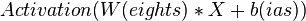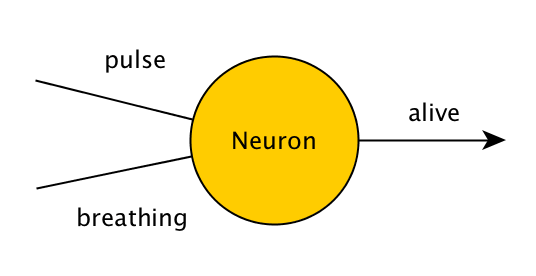# Artificial neural network facts for kids

Kids Encyclopedia Facts

A neural network (also called an ANN or an artificial neural network) is a sort of computer software, inspired by biological neurons. Biological brains are capable of solving difficult problems, but each neuron is only responsible for solving a very small part of the problem. Similarly, a neural network is made up of cells that work together to produce a desired result, although each individual cell is only responsible for solving a small part of the problem. This is one method for creating artificially intelligent programs.

Neural networks are an example of machine learning, where a program can change as it learns to solve a problem. A neural network can be trained and improved with each example, but the larger the neural network, the more examples it needs to perform well—often needing millions or billions of examples in the case of deep learning.

## Overview

There are two ways to think of a neural network. First is like a human brain. Second is like a mathematical equation.

A network starts with an input, somewhat like a sensory organ. Information then flows through layers of neurons, where each neuron is connected to many other neurons. If a particular neuron receives enough stimuli, then it sends a message to any other neuron is it connected to through its axon. Similarly, an artificial neural network has an input layer of data, one or more hidden layers of classifiers, and an output layer. Each node in each hidden layer is connected to a node in the next layer. When a node receives information, it sends along some amount of it to the nodes it is connected to. The amount is determined by a mathematical function called an activation function, such as sigmoid or tanh.

Thinking of a neural network like a mathematical equation, a neural network is simply a list of mathematical operations to be applied to an input. The input and output of each operation is a tensor (or more specifically a vector or matrix). Each pair of layers is connected by a list of weights. Each layer has several tensors stored in it. An individual tensor in a layer is called a node. Each node is connected to some or all of the nodes in the next layer by a weight. Each node also has a list of values called biases. The value of each layer is then the out of the activation function of the values of the current layer (called X) multiplied by the weights.$Activation(W(eights) * X + b(ias))$

A cost function is defined for the network. The loss function tries to estimate how well the neural network is doing at its assigned task. Finally, an optimization technique is applied to minimize the output of the cost function by changing the weights and biases of the network. This process is called training. Training is done one small step at a time. After thousands of steps, the network is typically able to do its assigned task pretty well.

## Example

Consider a program that checks whether a person is alive. It checks two things - the pulse, and breath.If a person has either a pulse or is breathing, the program will output 'alive', otherwise, it will output 'dead'. In a program that does not learn over time, this would be written as:

```function isAlive(pulse, breathing) {
if(pulse || breathing) {
return true;
} else {
return false;
}
}
```

A very simple neural network, made of just one neuron that solves the same problem will look like this:The values of pulse, breathing, and alive will be either 0 or 1, representing false and true. Thus, if this neuron is given the values (0,1), (1,0) or (1,1), it should output 1, and if it is given (0,0), it should output 0. The neuron does this by applying a simple mathematical operation to the input - it adds whatever values it has been given together, and then adds its own hidden value, which is called a 'bias'. To start with, this hidden value is random, and we adjust it over time if the neuron is not giving us the desired output.

If we add values such as (1,1) together, we might end up with numbers greater than 1, but we want our output to be between 0 and 1! To solve this, we can apply a function which limits our actual output to 0 or 1, even if the result of the neuron's math was not within the range. In more complicated neural networks, we apply a function (such as sigmoid) to the neuron, so that its value will be between 0 or 1 (such as 0.66), and then we pass on this value to the next neuron all the way until we need our output.

## Learning methods

There are three ways a neural network can learn: supervised learning, unsupervised learning and reinforcement learning. These methods all work by either minimizing or maximizing a cost function, but each one is better at certain tasks.

Recently, a research team from the University of Hertfordshire, UK used reinforcement learning to make an iCub humanoid robot learn to say simple words by babbling.Artificial neural network Facts for Kids. Kiddle Encyclopedia.